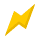120 Volts\$3,899.00/Each120 Volts\$2,559.00/Each120 Volts\$1,939.00/Each120 Volts\$3,189.00/Each120 Volts\$9,069.00/Each120 Volts\$2,879.00/Each120 Volts\$8,979.00/Each120 Volts\$5,039.00/Each

\$4,959.00/Each

\$14,339.00/Each

\$12,299.00/Each

\$4,789.00/Each

\$5,549.00/Each

\$5,889.00/Each120 Volts\$6,319.00/Each